# MUSE specific tools (mpdaf.MUSE)¶

## Python interface for MUSE slicer numbering scheme¶

The Slicer class contains a set of static methods to convert a slice number between the various numbering schemes. The definition of the various numbering schemes and the conversion table can be found in the “Global Positioning System” document (VLT-TRE-MUSE-14670-0657).

All the methods are static and thus there is no need to instantiate an object to use this class.

For example, we convert slice number 4 in CCD numbering to SKY numbering:

In : from mpdaf.MUSE import Slicer

In : Slicer.ccd2sky(4)
Out: 10


Now we convert slice number 12 of stack 3 in OPTICAL numbering to CCD numbering:

In : Slicer.optical2sky((2, 12))
Out: 25


## MUSE LSF models¶

Warning

LSF class is currently under development

Only one model of LSF (Line Spread Function) is currently available.

### LSF qsim_v1¶

This is a simple model where the LSF is supposed to be constant over the filed of view. It uses a simple parametric model of variation with wavelength.

The model is a convolution of a step function with a Gaussian. The resulting function is then sample by the pixel size:

LSF = T(y2+dy/2) - T(y2-dy/2) - T(y1+dy/2) + T(y1-dy/2)

T(x) = exp(-x**2/2) + sqrt(2*pi)*x*erf(x/sqrt(2))/2

y1 = (y-h/2) / sigma

y2 = (y+h/2) / sigma


The slit width is assumed to be constant (h = 2.09 pixels). The Gaussian sigma parameter is a polynomial approximation of order 3 with wavelength:

c = [-0.09876662, 0.44410609, -0.03166038, 0.46285363]

sigma(x) = c + c*x + c*x**2 + c*x**3


To use it, create a LSF object with attribute ‘typ’ equal to ‘qsim_v1’:

In : from mpdaf.MUSE import LSF

In : lsf = LSF(typ='qsim_v1')


Then get the LSF array by using get_LSF:

In : lsf_6000 = lsf.get_LSF(lbda=6000, step=1.25, size=11)

In : import matplotlib.pyplot as plt

In : import numpy as np

In : plt.plot(np.arange(-5,6), lsf_6000, drawstyle='steps-mid')
Out: [<matplotlib.lines.Line2D at 0x7fdd1d990ef0>]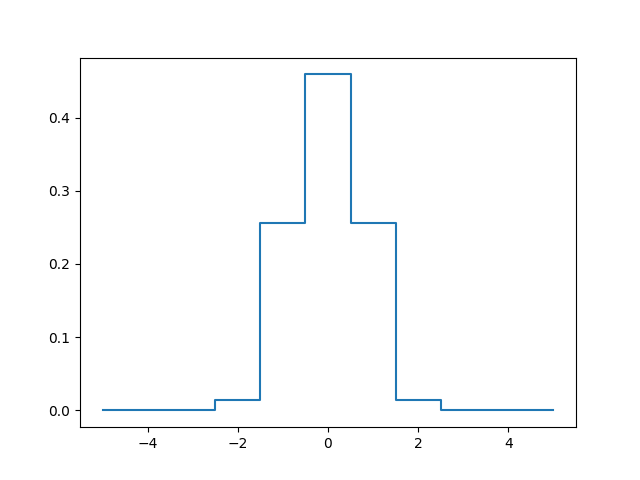## MUSE FSF models¶

Warning

FSF class is currently under development

Two models of FSF (Field Spread Function) are currently available:

### Example with MoffatModel2¶

The MUSE FSF is modelled as a Circular Moffat function with a FWHM and beta parameter which varies with the wavelength according to:

$$FWHM = p_0 \times l^n + p_1 \times l^{n-1} + p_n$$

$$\beta = k_0 \times l^m + k_1 \times l^{m-1} + k_m$$

$$l = (\lambda - \lambda_1) / (\lambda_2 - \lambda_1) - 0.5$$

With:

• $$p_i$$ polynomial coefficient for FWHM polynomial approximation

• n FWHM polynomial degre

• $$k_i$$ polynomial coefficient for beta polynomial approximation

• m beta polynomial degre

• $$\lambda_1$$,:math:lambda_2 reference wavelength for normalisation

We create the FSFModel object like this:

In : from mpdaf.MUSE import FSFModel, MoffatModel2

In : fsf = MoffatModel2(fwhm_pol=[-0.2, 0.7], beta_pol=[2.8], lbrange=[4800,9300], pixstep=0.2)

In : fsf.info()
[INFO] Wavelength range: 4800-9300
[INFO] FWHM Poly: [-0.2, 0.7]
[INFO] FWHM (arcsec): 0.80-0.60
[INFO] Beta Poly: [2.8]
[INFO] Beta values: 2.80-2.80


There are various way to create a fsf model, by fitting a point source or by using the external PSF reconstruction module muse_psfrec.

#### Using a star in the field of view¶

from mpdaf.obj import cube
cube = Cube('DATACUBE.fits')
ra,dec = (53.1583804, -27.7949048)
fsf = MoffatModel2.from_starfit(cube, (dec,ra), fwhmdeg=3, betadeg=2)

[INFO] FSF from star fit at Ra: 53.15838 Dec: -27.79491 Size 5.1 Nslice 20 FWHM poly deg 3 BETA poly deg 2
[debug] getting 20 images around object ra:-27.794905 dec:53.158380
[debug] -- first fit on white light image
[DEBUG] RA: 53.15837 DEC: -27.79492 FWHM 0.66 BETA 2.39 PEAK 276.5 BACK 0.2
[DEBUG] -- Second fit on all images
[DEBUG] 1 RA: 53.15837 DEC: -27.79491 FWHM 0.75 BETA 2.42 PEAK 174.6 BACK 0.1
[DEBUG] 2 RA: 53.15837 DEC: -27.79491 FWHM 0.75 BETA 2.45 PEAK 151.2 BACK 0.1
[DEBUG] ......
[DEBUG] 20 RA: 53.15837 DEC: -27.79493 FWHM 0.60 BETA 2.49 PEAK 293.2 BACK 0.2
[DEBUG] -- Third fit on all images
[DEBUG] -- Polynomial fit of BETA(lbda)
[DEBUG] BETA poly [ 0.07567152 -0.03087214  2.43062733]
[DEBUG] RA: 53.15837 DEC: -27.79491 FWHM 0.75 BETA 2.47 PEAK 174.0 BACK 0.1
[DEBUG] RA: 53.15837 DEC: -27.79491 FWHM 0.75 BETA 2.46 PEAK 151.1 BACK 0.1
[DEBUG] ......
[DEBUG] RA: 53.15837 DEC: -27.79493 FWHM 0.60 BETA 2.44 PEAK 294.2 BACK 0.2
[DEBUG] -- Polynomial fit of FWHM(lbda)
[DEBUG] FWHM poly [ 0.22042019  0.02317024 -0.21365627  0.66766788]
[DEBUG] -- return FSF model


The fsf.fit dictionary can be used to check the fit quality:

fig,ax = plt.subplots(1,2)
ax.plot(fsf.fit['wave'], fsf.fit['fwhmfit'], 'ok')
ax.plot(fsf.fit['wave'], fsf.fit['fwhmpol'], '-r')
ax.plot(fsf.fit['wave'], fsf.fit['betafit'], 'ok')
ax.plot(fsf.fit['wave'], fsf.fit['betapol'], '-r')
ax.set_ylim(2,3)


#### Using psfrec¶

If the MUSE observations have been done in GLAO WFM mode, then one can get a fsf model from the AO telemetry information saved in the raw data.

Warning

The psfrec module must be installed. Check https://muse-psfr.readthedocs.io for information.

fsf = MoffatModel2.from_psfrec('MUSE.2018-09-11T05:44:14.890.fits')

[DEBUG] Computing PSF from Sparta data file MUSE.2018-09-11T05:44:14.890.fits
[INFO] Processing SPARTA table with 13 values, njobs=-1 ...
[INFO] 6/13 : Using only 3 values out of 4 after outliers rejection
[INFO] Compute PSF with seeing=0.69 GL=0.64 L0=18.74
[INFO] Compute PSF with seeing=0.58 GL=0.48 L0=14.40
[INFO] Using three lasers mode
[INFO] Compute PSF with seeing=0.58 GL=0.49 L0=16.65
......
[INFO] Compute PSF with seeing=0.69 GL=0.46 L0=15.24
[INFO] Compute PSF with seeing=0.67 GL=0.56 L0=15.86
[DEBUG] 01: Seeing 0.69,0.69,0.70,0.69 GL 0.64,0.65,0.64,0.63 L0 18.34,19.04,17.48,20.07
[DEBUG] 02: Seeing 0.66,0.65,0.69,0.67 GL 0.57,0.56,0.56,0.54 L0 14.87,18.44,14.24,15.89
......
[DEBUG] 12: Seeing 0.67,0.67,0.66,0.65 GL 0.55,0.51,0.52,0.48 L0 9.37,11.07,10.19,13.05
[DEBUG] 13: Seeing 0.64,0.65,0.69,0.66 GL 0.57,0.56,0.55,0.53 L0 15.43,16.22,12.61,14.41
[DEBUG] Fitting polynomial on FWHM (lbda) and Beta(lbda)


If the MUSE observations have been done in GLAO WFM mode, then one can get a fsf model from the AO telemetry information saved in the raw data.

Warning

The psfrec module must be installed. Check https://muse-psfr.readthedocs.io for information.

### Methods¶

Various methods allow to get the FSF array (2D or 3D, as mpdaf Image or Cube) for given wavelengths, or the FWHM in pixel and in arcseconds.

In : lbda = np.array([5000, 9000])

In : fsf.get_fwhm(lbda)
Out: array([0.79111111, 0.61333333])

In : fsf.get_fwhm(lbda, unit='pix')
Out: array([3.95555556, 3.06666667])

In : im5000 = fsf.get_2darray(lbda, shape=(25, 25))

In : fsfcube = fsf.get_3darray(lbda, shape=(25, 25))

In : plt.figure()
Out: <Figure size 640x480 with 0 Axes>

In : plt.imshow(im5000)
Out: <matplotlib.image.AxesImage at 0x7fdd1bb62160>

In : plt.figure()
Out: <Figure size 640x480 with 0 Axes>

In : plt.imshow(fsfcube)
Out: <matplotlib.image.AxesImage at 0x7fdd1da3cc88>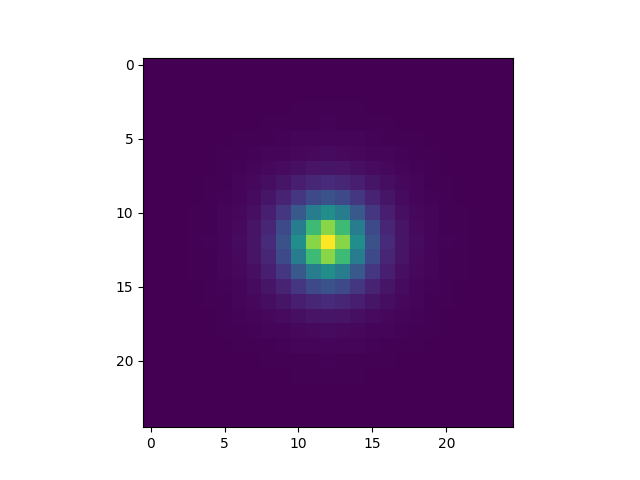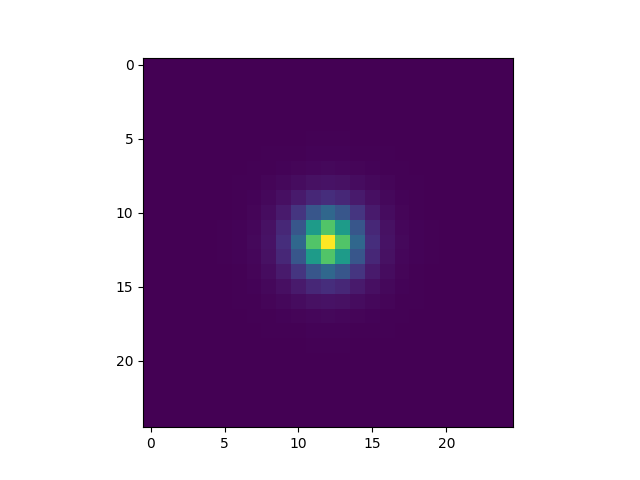The FSF model can be saved to a FITS header with mpdaf.MUSE.FSFModel.to_header, and read with mpdaf.MUSE.FSFModel.read.

## MUSE mosaic field map¶

Warning

FieldsMap class is currently under development

FieldsMap reads the possible FIELDMAP extension of the MUSE data cube.

In : from mpdaf.MUSE import FieldsMap

In : fmap = FieldsMap('sdetect/subcub_mosaic.fits', extname='FIELDMAP')


get_pixel_fields returns a list of fields that cover a given pixel (y, x):

In : fmap.get_pixel_fields(0,0)
Out: ['UDF-06', 'UDF-09']

In : fmap.get_pixel_fields(20,20)
Out: ['UDF-06']


get_field_mask returns an array with non-zeros values for pixels matching a field:

In : plt.figure()
Out: <Figure size 640x480 with 0 Axes>

Out: <matplotlib.image.AxesImage at 0x7fdd1bd559e8>

In : plt.figure()
Out: <Figure size 640x480 with 0 Axes>

Out: <matplotlib.image.AxesImage at 0x7fdd1c985a58>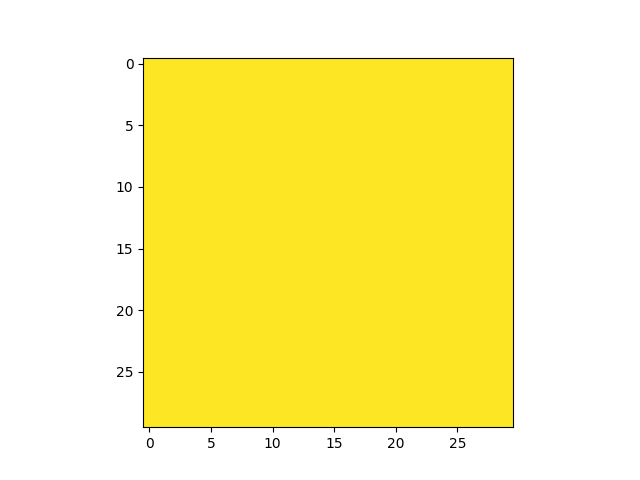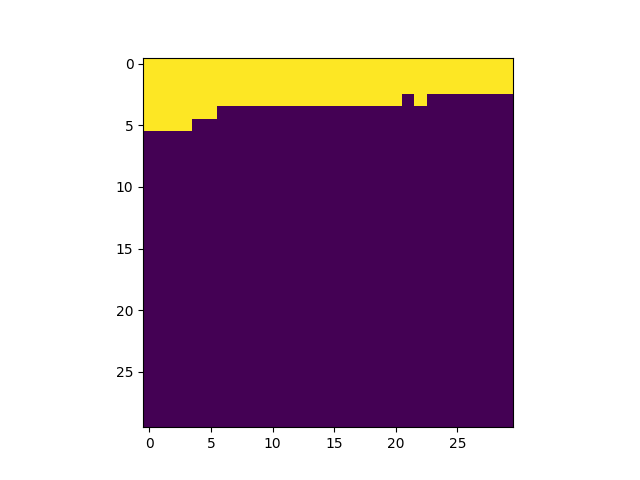## Reference/API¶

### mpdaf.MUSE Package¶

#### Functions¶

 FSF([typ]) This class offers Field Spread Function (FSF) models for MUSE. Moffat2D(fwhm, beta, shape[, center, normalize]) Compute Moffat for a value or array of values of FWHM and beta. combine_fsf(fsflist[, nlbda, size]) Combine a list of FSF create_psf_cube(shape, fwhm[, beta, wcs, …]) Create a PSF cube with FWHM varying along each wavelength plane. get_FSF_from_cube_keywords(cube, size) Return a cube of FSFs corresponding to the keywords presents in the MUSE data cube primary header (‘FSF***’)

#### Classes¶

 Base class for FSF models. FieldsMap([filename, nfields]) Class to work with the mosaic field map. LSF([typ]) This class offers Line Spread Function models for MUSE. MoffatModel2(fwhm_pol, beta_pol, lbrange, …) Circular MOFFAT beta=poly(lbda) fwhm=poly(lbda). OldMoffatModel(a, b, beta, pixstep[, field]) Moffat FSF with fixed beta and FWHM varying with wavelength. Slicer Convert slice number between the various numbering schemes.